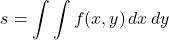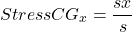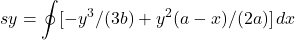# Foundation Bearing Pressures using Jupyter and PyXLL (with videos) Mar 2023

Check out the videos below
May 2 2023 Update

Since early March, off and on (mostly on 😊), I have been working on improvements to this application. The iterative method is exposed to a surprising number of corner cases; I offer a short summary below.

Limitations and corner cases
TERMINOLOGY

• Polygon: defines the extent of the base of the foundation
• Load point (LP): defines the point of application of the vertical load
• Center of gravity (CG): defines the CG of a polygon
• Neutral axis (NA): defines the line of zero stress for the reduced polygon
Simple example of a rectangular footing showing reduced bearing area
• Reduced polygon: defines the effective portion of the base of the foundation
INPUT

The user enters:

• the coordinates of a polygon, in clockwise or counterclockwise order
METHOD

The method of analysis is iterative.

The iterative method requires that the polygon be located in the first quadrant of the plane. The program takes care of that internally; the user can locate the polygon on any XY coordinate system.

The CG of the input polygon is calculated.

Within the polygon, the iterative procedure will tend to converge if the LP is such thatthat is, if the LP is lower left of the CG.

Internally, therefore, the program must rearrange the coordinate system to place the load point in the as-needed position. Turns out there are 8 possible transformations for an XY coordinate system (right click image to see in separate tab).OUTPUT

After the iteration is complete, the program returns:

• the input polygon
• the input polygon CG
• the input polygon area
• the neutral axis of the reduced polygon
• the reduced polygon
• the reduced polygon CG
• the reduced polygon area
• the reduced area ratio
RESULTS

Hover over chart elements to see analysis results.
Click legend elements to toggle on an off.
Static Charts (right click chart to see in separate tab)Foundation Bearing Pressures
I built a Jupyter notebook to calculate foundation bearing pressures.

My Jupyter notebook can be used stand-alone or in conjunction with PyXLL.

Here are a few of the features.

• The footing is defined using a simple list of 2D coordinates, a closed polygon
• The single concentrated loading point is defined using simple 2d coordinates, a single point
• I built a Point class to allow easy manipulation of 2D coordinates
• I built a Points class to allow easy manipulation of lists of 2D coordinates
• I built a Section class with methods for determining and manipulating section properties such as determining the resultant polygon after a given line (neutral axis) makes multiple intersections in a given polygon (section perimeter).
• I built a Solver function and a Plot function to simplify model analysis (one line for each function)
• I used Plotly for my plot routine
• The as-built foundation is plotted: coordinates, area and CG are shown
• The effective foundation is plotted: coordinates, area and CG are shown
• The neutral axis is shown
• The load point is shown
• The resultant stress are shown at each vertex
• PyXLL displays a static image of the Plotly chart
• The static image is displayed below the function call
• An optional LIVE Plotly HTML chart is built (see function call below)
• The optional LIVE Plotly HTML chart may be viewed using an Excel hyperlink
• Allows verification of all geometry
• For extra clarity, all plot items can be toggled
• The Excel function call is a one liner:
#

[PYTHON]
plot_width=640, plot_height=640,

[EXCEL]
plot_width, plot_height,

#

• Lpts is a 2D named range
(these are the X, Y coordinates of the polygon)
• plot_width is an optional integer input
• plot_height is an optional integer input
• pad_ratio is an optional float input
(allows padding around the plotted polygon)
• live_plotQ is an optional boolean input

Click for example live Chart! — hover over load point, vertices, CGs.

The plot elements can be toggled off and on (click on the legend). The plot can be panned and zoomed.

References

1) My calculations are based on an article by Eli Czerniak.

Computer Foundation Design
“How to Calculate Footing Soil Bearing by Computer”
El Czerniak, Fluor Corporation
Gulf Publishing Company, Houston, Texas
1963

This was a great article 60 years ago, and remains so well into the 21st century.

2) The excellent article by Dr. Drang presenting the use of line integrals to calculate section properties. In my Point class, I used the basic functions as given by Dr. Drang with very slight modifications. I added a couple functions for my specific calculations.

https://leancrew.com/all-this/2018/01/greens-theorem-and-section-properties/

For example, here is my trial_fxy method; this method uses line integrals to determine the ‘CG’ of the uniformly varying stress on the effective area of the foundation. With quite a bit of help from Sympy 😊, I built this function to check my results. The neutral axis and the associated dimensions a and b are shown in the reference diagram below.Using area integrals:Therefore, using line integrals:The Python code:


class Section():

def trial_fxy(pts, a, b):
'''Input: polygon as list of points, pts
Neutral axis x-intercept, a
Neutral axis y-intercept, b
points are type Point
Output:
'''
# polygon must be a 'closed' set of coordinates
# therefore, the last point must equal the first point

if pts != pts[-1]:
pts = pts + pts[:1]

x = [ c.x for c in pts ]
y = [ c.y for c in pts ]

sx = sy = sx1 = sy1 = sk = 0

for i in range(len(pts) - 1):
x0 = x[i]
x1 = x[i+1]
y0 = y[i]
y1 = y[i+1]

s = ((-2*a*b*x0*y0 + 2*a*b*x1*y0 +
a*x0*y0**2 - a*x1*y0**2 + 2*b*x0**2*y0 -
2*b*x0*x1*y0)/(2*a*b) +

(a*x0*y0**2 -
2*a*x0*y0*y1 + a*x0*y1**2 -
a*x1*y0**2 + 2*a*x1*y0*y1 - a*x1*y1**2 +
2*b*x0**2*y0 - 2*b*x0**2*y1 -
4*b*x0*x1*y0 + 4*b*x0*x1*y1 + 2*b*x1**2*y0 -
2*b*x1**2*y1)/(6*a*b) +

(a*b*x0*y0 - a*b*x0*y1 - a*b*x1*y0 +
a*b*x1*y1 - a*x0*y0**2 + a*x0*y0*y1 +
a*x1*y0**2 - a*x1*y0*y1 - 2*b*x0**2*y0 +
b*x0**2*y1 + 3*b*x0*x1*y0 -
b*x0*x1*y1 - b*x1**2*y0)/(2*a*b))

ssxx = ((-2*a*b*x0**2*y0 + 2*a*b*x0*x1*y0 +
a*x0**2*y0**2 - a*x0*x1*y0**2 +
2*b*x0**3*y0 - 2*b*x0**2*x1*y0)/(2*a*b) +

(4*a*b*x0**2*y0 - 2*a*b*x0**2*y1 -
6*a*b*x0*x1*y0 + 2*a*b*x0*x1*y1 +
2*a*b*x1**2*y0 - 3*a*x0**2*y0**2 +
2*a*x0**2*y0*y1 + 4*a*x0*x1*y0**2 -
2*a*x0*x1*y0*y1 - a*x1**2*y0**2 -
6*b*x0**3*y0 + 2*b*x0**3*y1 +
10*b*x0**2*x1*y0 - 2*b*x0**2*x1*y1 -
4*b*x0*x1**2*y0)/(4*a*b) +

(-a*x0**2*y0**2 + 2*a*x0**2*y0*y1 -
a*x0**2*y1**2 + 2*a*x0*x1*y0**2 -
4*a*x0*x1*y0*y1 + 2*a*x0*x1*y1**2 -
a*x1**2*y0**2 + 2*a*x1**2*y0*y1 -
a*x1**2*y1**2 - 2*b*x0**3*y0 +
2*b*x0**3*y1 + 6*b*x0**2*x1*y0 -
6*b*x0**2*x1*y1 - 6*b*x0*x1**2*y0 +
6*b*x0*x1**2*y1 + 2*b*x1**3*y0 -
2*b*x1**3*y1)/(8*a*b) +

(-2*a*b*x0**2*y0 +
2*a*b*x0**2*y1 + 4*a*b*x0*x1*y0 -
4*a*b*x0*x1*y1 - 2*a*b*x1**2*y0 +
2*a*b*x1**2*y1 + 3*a*x0**2*y0**2 -
4*a*x0**2*y0*y1 + a*x0**2*y1**2 -
5*a*x0*x1*y0**2 + 6*a*x0*x1*y0*y1 -
a*x0*x1*y1**2 + 2*a*x1**2*y0**2 -
2*a*x1**2*y0*y1 + 6*b*x0**3*y0 -
4*b*x0**3*y1 - 14*b*x0**2*x1*y0 +
8*b*x0**2*x1*y1 + 10*b*x0*x1**2*y0 -
4*b*x0*x1**2*y1 - 2*b*x1**3*y0)/(6*a*b))

ssyy = ((-3*a*b*x0*y0**2 + 3*a*b*x1*y0**2 +
2*a*x0*y0**3 - 2*a*x1*y0**3 +
3*b*x0**2*y0**2 -
3*b*x0*x1*y0**2)/(6*a*b) +

(2*a*b*x0*y0**2 - 2*a*b*x0*y0*y1 -
2*a*b*x1*y0**2 + 2*a*b*x1*y0*y1 -
2*a*x0*y0**3 + 2*a*x0*y0**2*y1 +
2*a*x1*y0**3 - 2*a*x1*y0**2*y1 -
3*b*x0**2*y0**2 + 2*b*x0**2*y0*y1 +
4*b*x0*x1*y0**2 - 2*b*x0*x1*y0*y1 -
b*x1**2*y0**2)/(4*a*b) +

(-2*a*x0*y0**3 +
6*a*x0*y0**2*y1 - 6*a*x0*y0*y1**2 +
2*a*x0*y1**3 + 2*a*x1*y0**3 -
6*a*x1*y0**2*y1 + 6*a*x1*y0*y1**2 -
2*a*x1*y1**3 - 3*b*x0**2*y0**2 +
6*b*x0**2*y0*y1 - 3*b*x0**2*y1**2 +
6*b*x0*x1*y0**2 - 12*b*x0*x1*y0*y1 +
6*b*x0*x1*y1**2 - 3*b*x1**2*y0**2 +
6*b*x1**2*y0*y1 -
3*b*x1**2*y1**2)/(24*a*b) +

(-a*b*x0*y0**2 + 2*a*b*x0*y0*y1 -
a*b*x0*y1**2 + a*b*x1*y0**2 -
2*a*b*x1*y0*y1 + a*b*x1*y1**2 +
2*a*x0*y0**3 - 4*a*x0*y0**2*y1 +
2*a*x0*y0*y1**2 - 2*a*x1*y0**3 +
4*a*x1*y0**2*y1 - 2*a*x1*y0*y1**2 +
3*b*x0**2*y0**2 - 4*b*x0**2*y0*y1 +
b*x0**2*y1**2 - 5*b*x0*x1*y0**2 +
6*b*x0*x1*y0*y1 - b*x0*x1*y1**2 +
2*b*x1**2*y0**2 - 2*b*x1**2*y0*y1)/(6*a*b))

sx += ssxx
sy += ssyy
sk += s

return sx/sk, sy/sk


What is PyXLL-Jupyter?

Integration for Jupyter notebooks and Microsoft Excel

https://github.com/pyxll/pyxll-jupyter

PyXLL to Jupyter Interface (with video) Dec 2020

What is PyXLL?